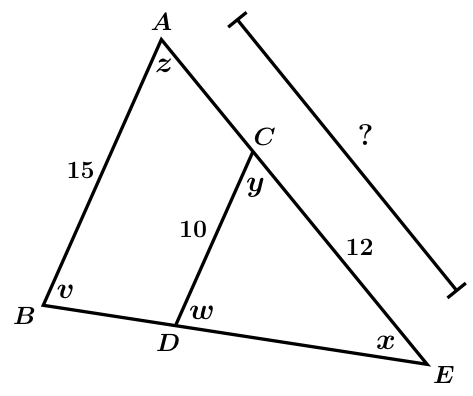# GEOM 3 | Lesson 3 | Practice 2Work on the following problems to apply similarity theorems and solve for unknown values. BEFORE you solve, state by which similarity theorem, IF ANY, you know the two triangles are similar by:

a) Set up the proportion and solve for $$x$$.b) What is the length of $$DE$$?c) Given that $$y= z$$ and $$w = v$$, find the length of $$AE$$ ?d) Solve each for $$x$$. (Find the value of $$x$$.)e) Solve for $$x$$.